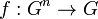Multiary semigroup

(diff) ← Older revision | Latest revision (diff) | Newer revision → (diff)
A multiary semigroup, also called a polyadic semigroup, is a$n$-ary semigroup for some$n \ge 2$. Note that the$n = 2$ case corresponds to the usual notion of semigroup.
A$n$-ary semigroup is defined as a set$G$ with a$n$-ary operation, i.e., a map$f: G^n \to G$ such that all different ways of associating expressions involving the$n$-ary operation$f$ yield equivalent results. Note that this boils down to checking that all the$n$ distinct possible ways of associating an expression of length$2n - 1$ give the same answer.